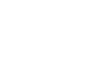State Boyle-s law- Charle-s law- Gay lusacc law or simply, state all the gas laws - letsdiskussankit shukla

merchant navy | Posted on | Science-Technology

State Boyle-s law- Charle-s law- Gay lusacc law or simply, state all the gas laws

0
0

Student of PCM in Vishwa Bharati Public School | Posted on

Boyle's Law

This law was given by Robert Boyle. It states that at constant temperature, the product of the pressure and volume of a given mass of an ideal gas in a closed system is always constant. Alternatively we can also state this law as the volume of a given mass of a fixed amount of gas is inversely related to pressure when the temperature is constant.

Algebrically :

Vα 1/P

V=k.1/P

PV=k

Or

P1V1=P2V2

Where,

P- pressure of gas

V - volume of gas

K- proportionality constant.

Charle's Law :

Charle's law also known as the law of volumes was given by Jacques Charles . This law states that for a given mass of an ideal gas at constant pressure , the volume is directly proportional to its Absolute Temperature assuming in a closed system .

Algebraically :

V α T

V = kT

V/T = K or

V1/T1=V2/T2

Where,

V- volume of gas

T - Absolute Temperature

K - proportionality constant

Gay-lussac's Law :

Gay lussac's law also known as Amontons law or the pressure law was given by Joseph Louis Gay Lussac. This law states that for a given mass and constant volume of an ideal gas the pressure exerted on the sides of its container is directly proportional to its Absolute Temperature.

Mathematically :

PαT

P = kT

P/T = k

P1/T1 = P2/T2

Where,

P - pressure of gas

T - Absolute Temperature

K - proportionality constant

This law states that volume occupied by an ideal gas is directly proportional to the number of molecules of the gas present in the container.

Mathematically:

V1/n1 = V2/n2

Where,

n- number of molecules of gas.

Combined Gas Law :

Emile Clapeyron combined Boyle's law Charles law gay-lussac's law along with Avogadro's law to give combined gas law which is also known as ideal gas law. This law gives relationship between the volume pressure and temperature for the fixed mass of a gas.

Mathematically :

PV = nRT

Where,

P is pressure

V is volume

n is number of moles

R is universal gas constant

T is temperature

value of R is 8.314 (kPa-L) / (mol-k).

This equation is exactly for an ideal gas which neglect various intermolecular effects.

This law has some important consequences which are as follows:

1. If temperature and pressure are kept constant , then volume of gas is directly proportional to the number of molecules of the gas.

2. If the temperature and volume remains constant then , the pressure of the gas is directly proportional to the number of molecules of the gas.

3. If the temperature and the number of molecules off the gas remain constant then the pressure is inversely proportional to the volume.

4. If the temperature changes and the number of gas molecules are kept constant then either pressure or volume or both will be directly proportional to the temperature.Graham's law :

This law states that the rate at which the number of molecules diffuse is inversely proportional to the square root of its density at constant temperature.

Dalton's law of partial pressure :

This law states that the pressure of a mixture of gases is simply the sum of partial pressures of the individual components.

Mathematically :

P(total) = P1 + P2 + P3 + ...........+Pn

Amagat's Law of partial pressure :

This law states that the volume of a mixture of gases ( or the volume of a container ) simply is sum of partial volumes of the individual components.

Mathematically :

V( total) = V1 + V2 + V3 + ..............+ Vn

Where V(total) is the total volume of the container .

V1 is the partial volume of the gas in the gas mixture at that pressure and that temperature

Henry's Law :

At the constant temperature the amount of gas dissolved in the given type and volume of the liquid is directly proportional to the partial pressure of the gas in equilibrium with the liquid.

P = kH.c

0
0